# Vectors, Complex Numbers and Mechanics | The Ultimate Guide to NESA’s Maths Reference Sheet

Make the most of NESA Maths Reference Sheet and test your maths skills with these sample questions and explanations for vectors, complex numbers and mechanics!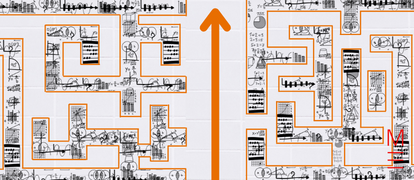The NESA Maths Reference Sheet is a great resource… if you know how to use it! Navigate vectors, complex numbers and mechanics with our Ultimate NESA Maths Reference Sheet Guide.

While memorisation has its place in learning, Matrix recommends that students learn to derive their responses and learn how to apply these formulae correctly. As a bonus, we’ve included a nifty HSC Maths Cheatsheet for you to download and print out!A free pocket-sized Maths handbook, so you're prepared.

All the key Maths formulas you need to revise, in one foldable cheatsheet.

Click on the following formulas to see what they mean and apply them to a practice question!

 Vectors $$|{\bf u}|=|x{\bf i}+y{\bf j}|=\sqrt{x^2+y^2}$$ $${\bf u} \cdot {\bf v} = |{\bf u}||{\bf v}|\text{cos} \theta = x_1 x_2 + y_1 y_2 ,$$ \begin{align*} \text{where } {\bf u} &= x_1 {\bf i} + y_1{\bf j}\\ \text{and } {\bf v} &= x_2{\bf i} + y_2{\bf j}\\ \end{align*} $${\bf r}={\bf a} + λ {\bf b}$$

Maths Extension 2 only:

## Vectors

 Use Formula Explanation Magnitude of the vector $$|{\bf u}|=|x{\bf i}+y{\bf j}|=\sqrt{x^2+y^2}$$ \begin{align*} |{\bf u}| &= \text{length/magnitude of }{\bf u} \\ (x, \ y) &= \text{ Cartesian coordinates of position vector } {\bf u} \\ {\bf i}&: \text{ unit vector in the direction of the x-axis} \\ {\bf j}&: \text{ unit vector in the direction of the y-axis} \\ \end{align*}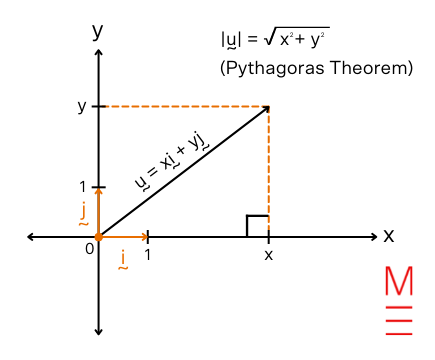Dot product $${\bf u} \cdot {\bf v} = |{\bf u}||{\bf v}|\text{cos} \theta = x_1 x_2 + y_1 y_2 ,$$\begin{align*} \text{where } {\bf u} &= x_1 {\bf i} + y_1{\bf j}\\ \text{and } {\bf v} &= x_2{\bf i} + y_2{\bf j}\\ \end{align*}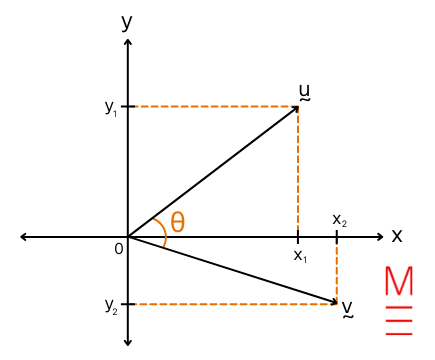Vector form of a line $${\bf r}={\bf a} + λ {\bf b}$$ A line can be expressed in many different forms like Cartesian form ($$y =mx+c$$) and vector form. When we express line $${\bf r}$$ in vector form, we describe it using a point $${\bf a}= \binom{x}{y}$$ that it passes through and its gradient vector $${\bf b}$$. By substituting different constant values for $$λ∈R$$, the vector equation $${\bf r}={\bf a} + λ {\bf b}$$ describes every single point on line $${\bf r}$$.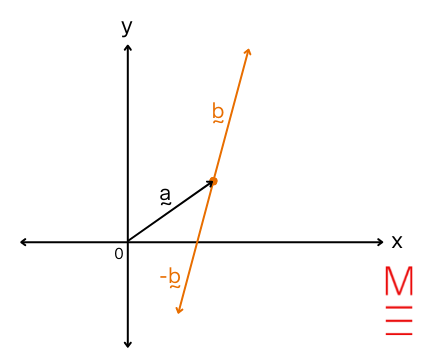Example 28:

Express the line y = 4x + 3 in vector form.

Solution 28:

 Find a point that y = 4x + 3 passes through: \begin{align*} \text{Substitute }x&=1 \text{ into } y=4x+3: \\ y &= (4)(1) + 3 \\ &= 7 \\ ∴ \text{A point on y = 4x + 3} &=  \binom{1}{7} \text{  (i.e. x = 1 and y = 7, representing the co-ordinate (1, 7)} \\ \end{align*}   Find a gradient vector of the line y = 4x + 3: Since the equation of the line is in the form y = mx + c, the gradient is the coefficient of x. \begin{align*} ∴ \text{Gradient} &=  4 \\ ⇒ \text{Gradient vector} &=  \binom{run}{rise} \\ &= \binom{1}{4}\end{align*} Now that we know that the line passes through $$\binom{1}{7}$$ and has a gradient vector of $$\binom{1}{4}$$, we can express the line in vector form: $${\bf r}= \binom{1}{7} + λ \binom{1}{4}$$ where $$λ∈R$$

Maths Extension 2 only:

## Complex Numbers

 Use Formula Explanation Cartesian form $$z=a+ib$$ $$z$$ is a complex number that can be expressed in many different forms. \begin{align*} a &= \text{x-coordinate of z} \\ b &= \text{y-coordinate of z} \\ \\ r &= |z| = \text{modulus or length of z} \\ \theta &= \text{argument or direction of z} \\ \end{align*}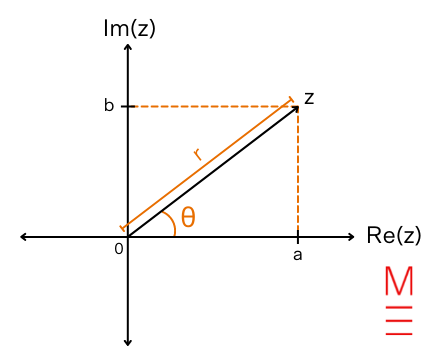Polar form $$z=r( \text{cos} \theta +i \text{sin} \theta)$$ Euler’s formula $$z=re^{i \theta}$$ De Moivre’s Theorem \begin{align*} [r( \text{cos} \theta + i \text{sin} \theta )]^n &= r^n (\text{cos} n \theta + i \text{sin} n \theta)\\ &= r^n e^{in \theta}\\ \end{align*} The argument of $$z^n$$ is n times as large as the argument of $$z$$ . i.e. $$Arg(z^n)= \text{n}Arg(z)$$ The modulus of $$z^n$$ is equal to the modulus of $$z$$ to the power of $$n$$ . i.e. $$|z^n|= |z|^n$$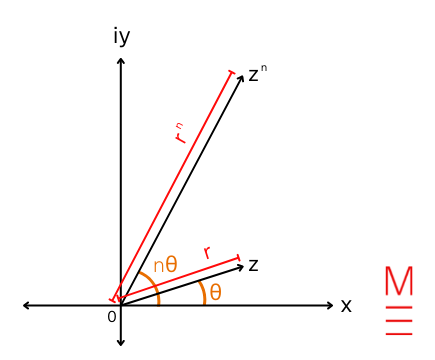## Mechanics

 Use Formula Explanation Manipulating expressions for acceleration, velocity and displacement $$\frac{d^2x}{dt^2} = \frac{dv}{dt} = v \frac{dv}{dx} = \frac{d}{dx} \left( \frac{1}{2} v^2 \right)$$ Need help understanding differentiation? See the following Maths Guides! Maths Adv Applications of differentiation Maths Ext 1 Applications of differentiation (Year 11) Differential equations Expression for displacement $$x$$ in terms of time $$t$$ $$x=a \text{cos} (nt+α)+c$$ \begin{align*} x & = \text{displacement} \\ \\ \ \frac{2\pi}{n} &= \text{Period} \\ a &= \text{Amplitude}\\ α &= \text{Phase shift}\\ c &= \text{Centre of motion} \end{align*} $$x=a \text{sin} (nt+α)+c$$ Expression for acceleration $$\ddot x$$ in terms of displacement $$x$$ $$\ddot x= -n^2(x-c)$$ $$\ddot x = \text{acceleration}$$

### Get free study tips and resources delivered to your inbox.

Join 75,893 students who already have a head start.

Our website uses cookies to provide you with a better browsing experience. If you continue to use this site, you consent to our use of cookies. Read our cookies statement.